FanDuel 2019 Championship Odds
+300
3 to 1Clippers
20.6% implied probability

+390
3.9 to 1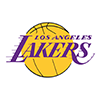Lakers
16.8% implied probability

+500
5 to 1Bucks
13.8% implied probability

+700
7 to 176ers
10.3% implied probability

+800
8 to 1Rockets
9.2% implied probability

+1600
16 to 1Nuggets
4.9% implied probability

+1800
18 to 1Jazz
4.3% implied probability

+2700
27 to 1Celtics
2.9% implied probability

+4200
42 to 1Raptors
1.9% implied probability

+4200
42 to 1Mavericks
1.9% implied probability

+5000
50 to 1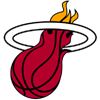Heat
1.6% implied probability

+5000
50 to 1Nets
1.6% implied probability

+6500
65 to 1Trail Blazers
1.3% implied probability

+6500
65 to 1Pacers
1.3% implied probability

+6500
65 to 1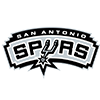Spurs
1.3% implied probability

No changes have been recorded yet.
+10000
100 to 1Pelicans
0.8% implied probability

+13000
130 to 1Timberwolves
0.6% implied probability

+16000
160 to 1Magic
0.5% implied probability

No changes have been recorded yet.
+16000
160 to 1Suns
0.5% implied probability

+18000
180 to 1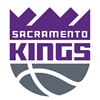Kings
0.5% implied probability

No changes have been recorded yet.
+21000
210 to 1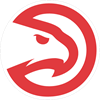Hawks
0.4% implied probability

No changes have been recorded yet.
+24000
240 to 1Pistons
0.3% implied probability

+25000
250 to 1Knicks
0.3% implied probability

No changes have been recorded yet.
+25000
250 to 1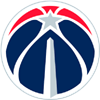Wizards
0.3% implied probability

No changes have been recorded yet.
+25000
250 to 1Thunder
0.3% implied probability

+25000
250 to 1Hornets
0.3% implied probability

No changes have been recorded yet.
+25000
250 to 1Grizzlies
0.3% implied probability

No changes have been recorded yet.
+25000
250 to 1Cavaliers
0.3% implied probability

No changes have been recorded yet.
+25000
250 to 1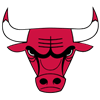Bulls
0.3% implied probability

+25000
250 to 1Warriors
0.3% implied probability